# NCERT Exemplar Class 12 Physics Solutions for Chapter 12 Atoms

NCERT Class 12 Physics Exemplar Solutions for Chapter 12 Atoms provide various questions which help students to comprehend and learn the topics quickly. This exemplar page has Class 12 Physics NCERT solved examples, MCQs, fill in the blanks, match the following and numerical problems.

Questions provided in exemplar solutions are essential, as some of them may be asked in the CBSE Class 12 examination and national-level entrance examinations like JEE Main, NEET and JEE Advanced.

## Download the PDF of the NCERT Exemplar for Class 12 Physics Chapter 12 Atoms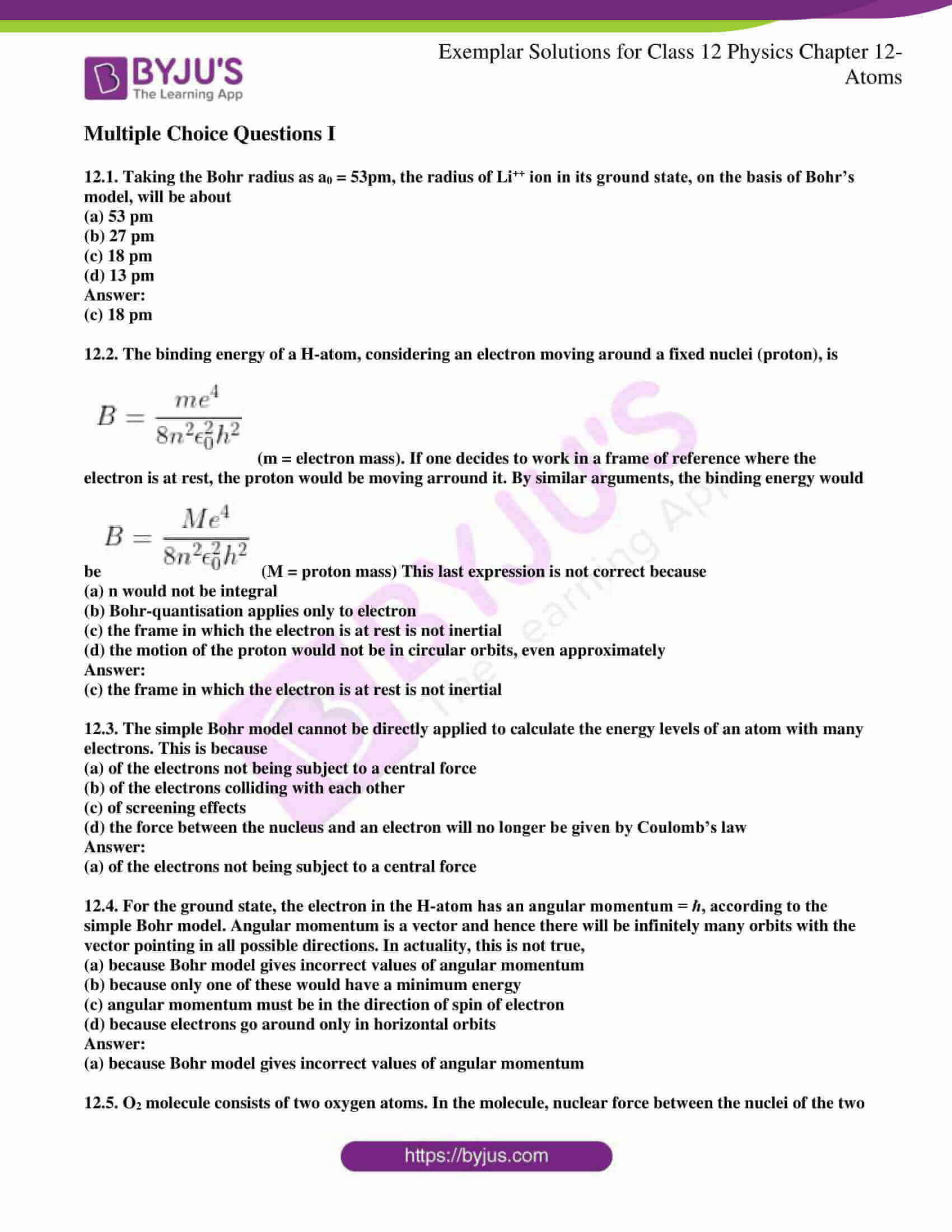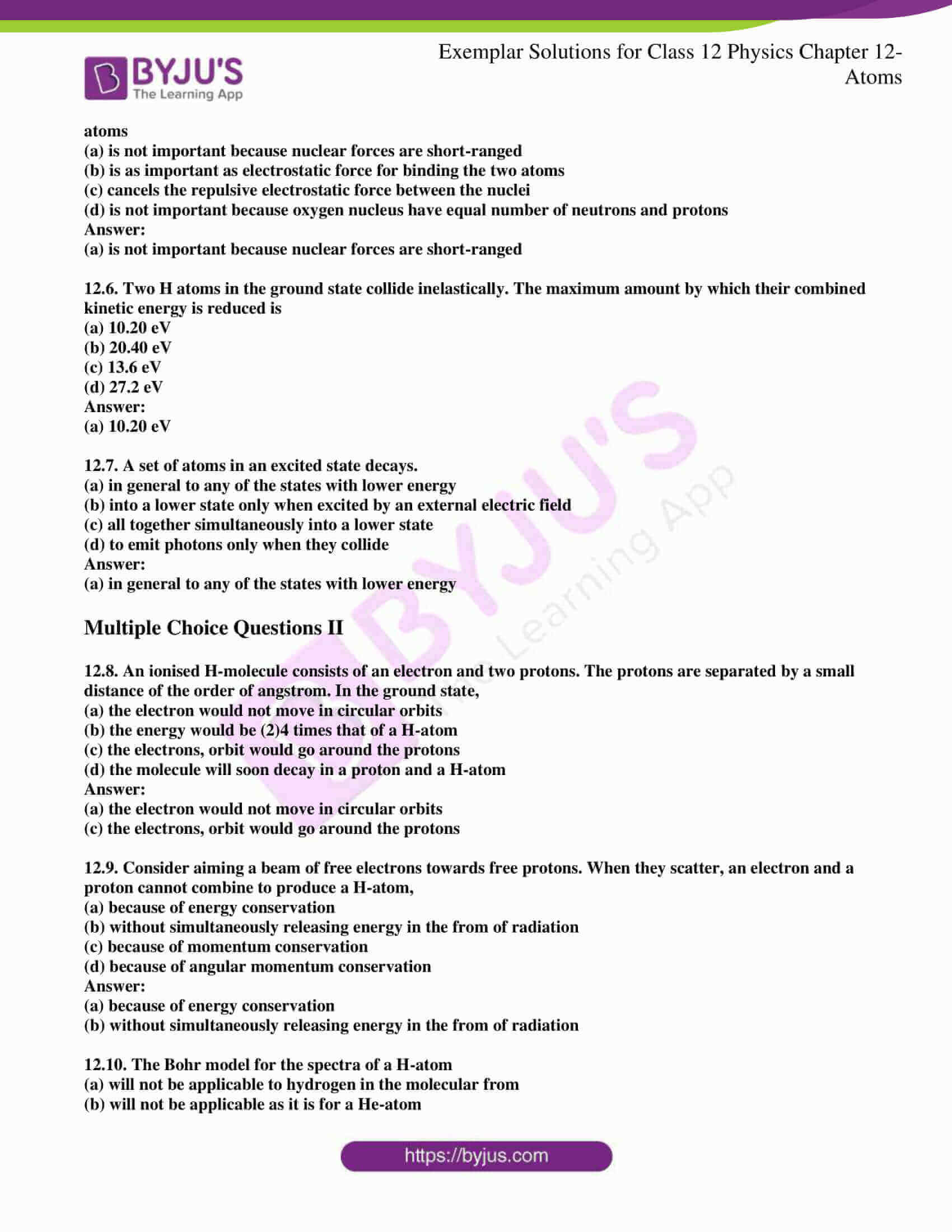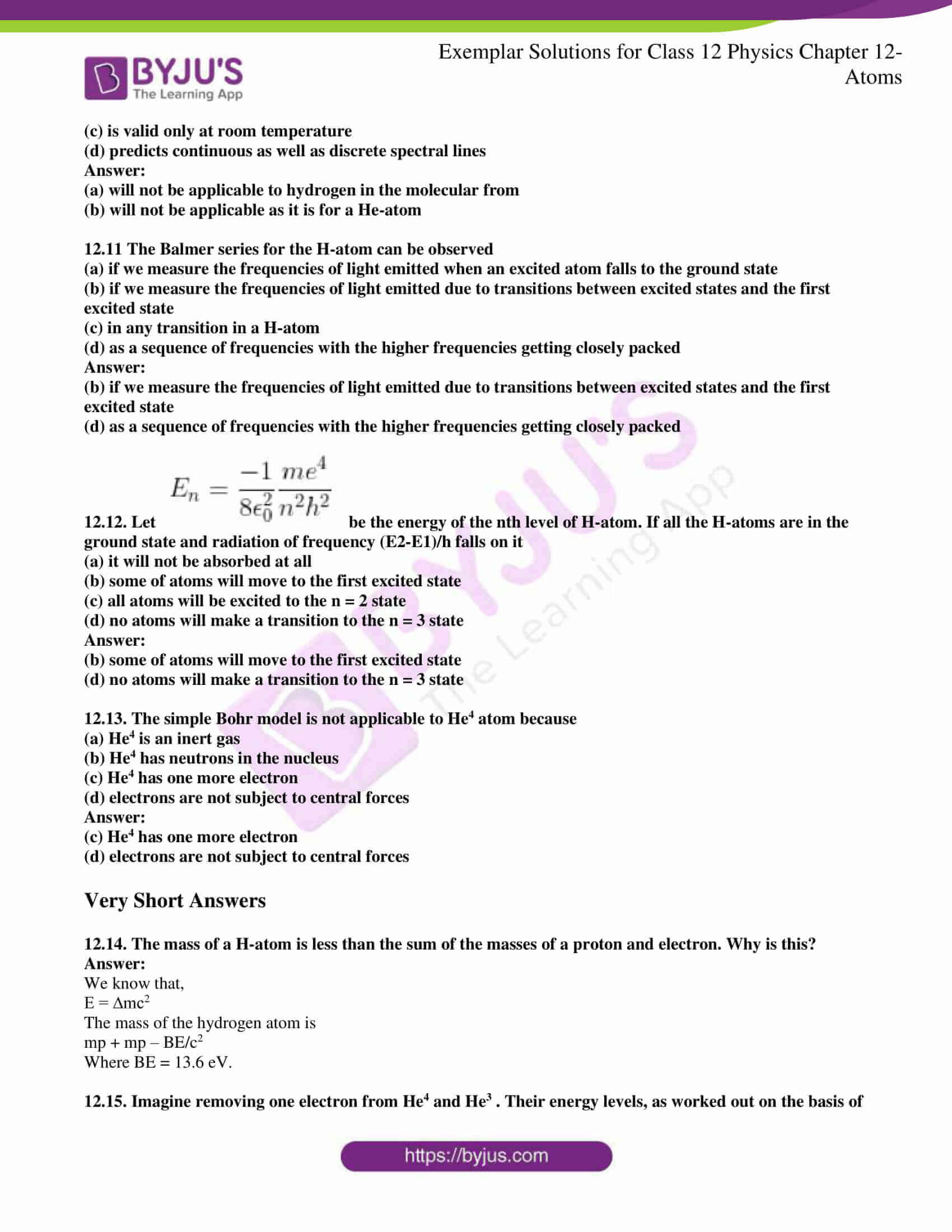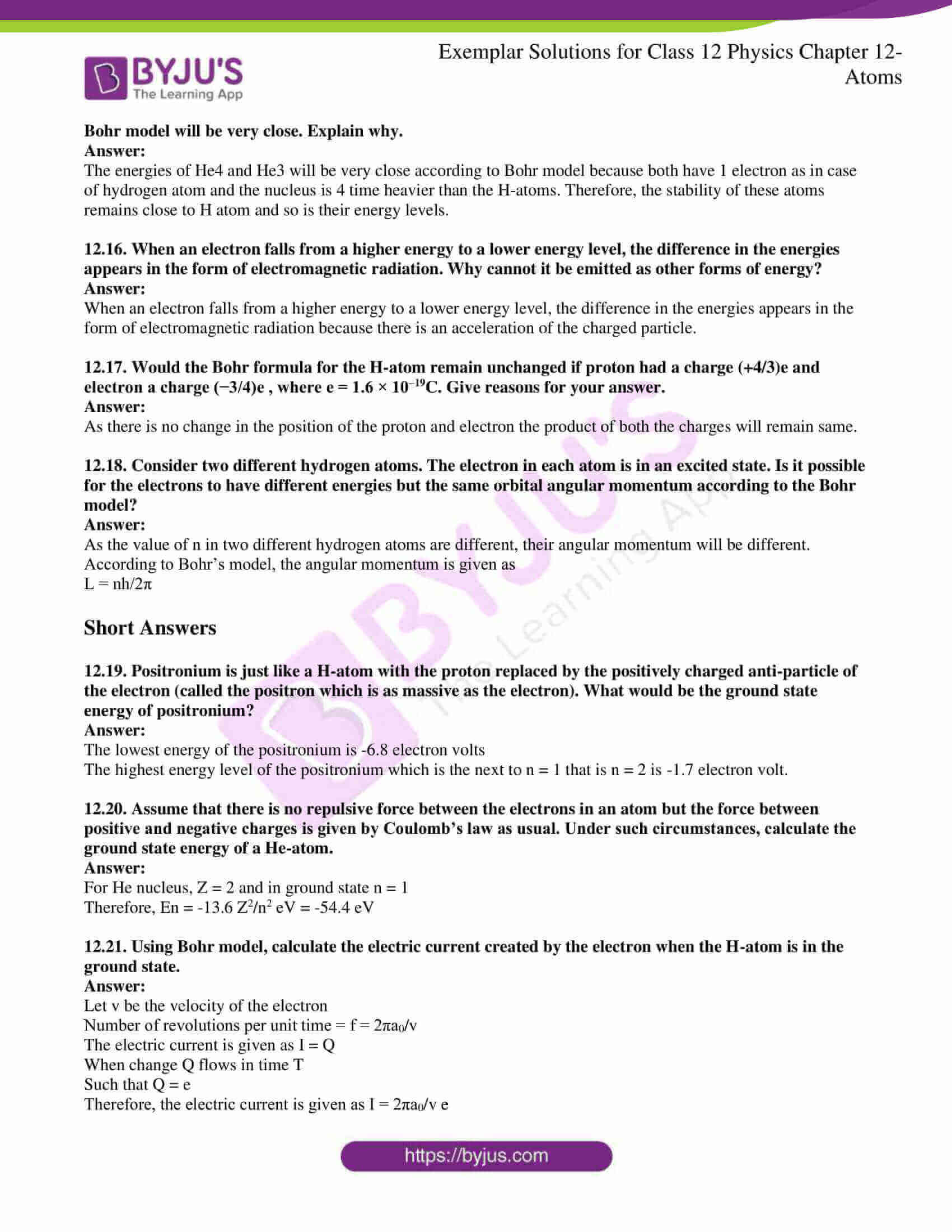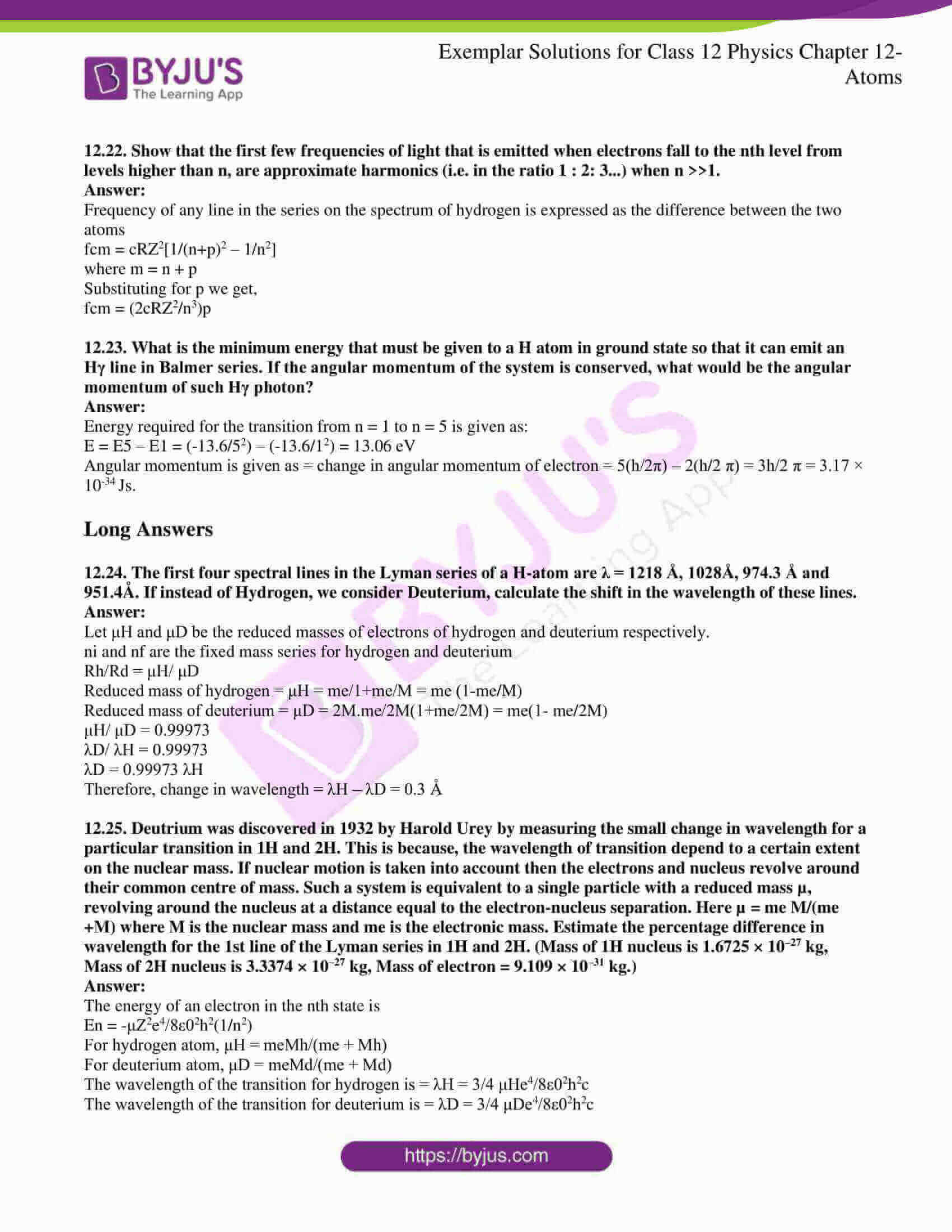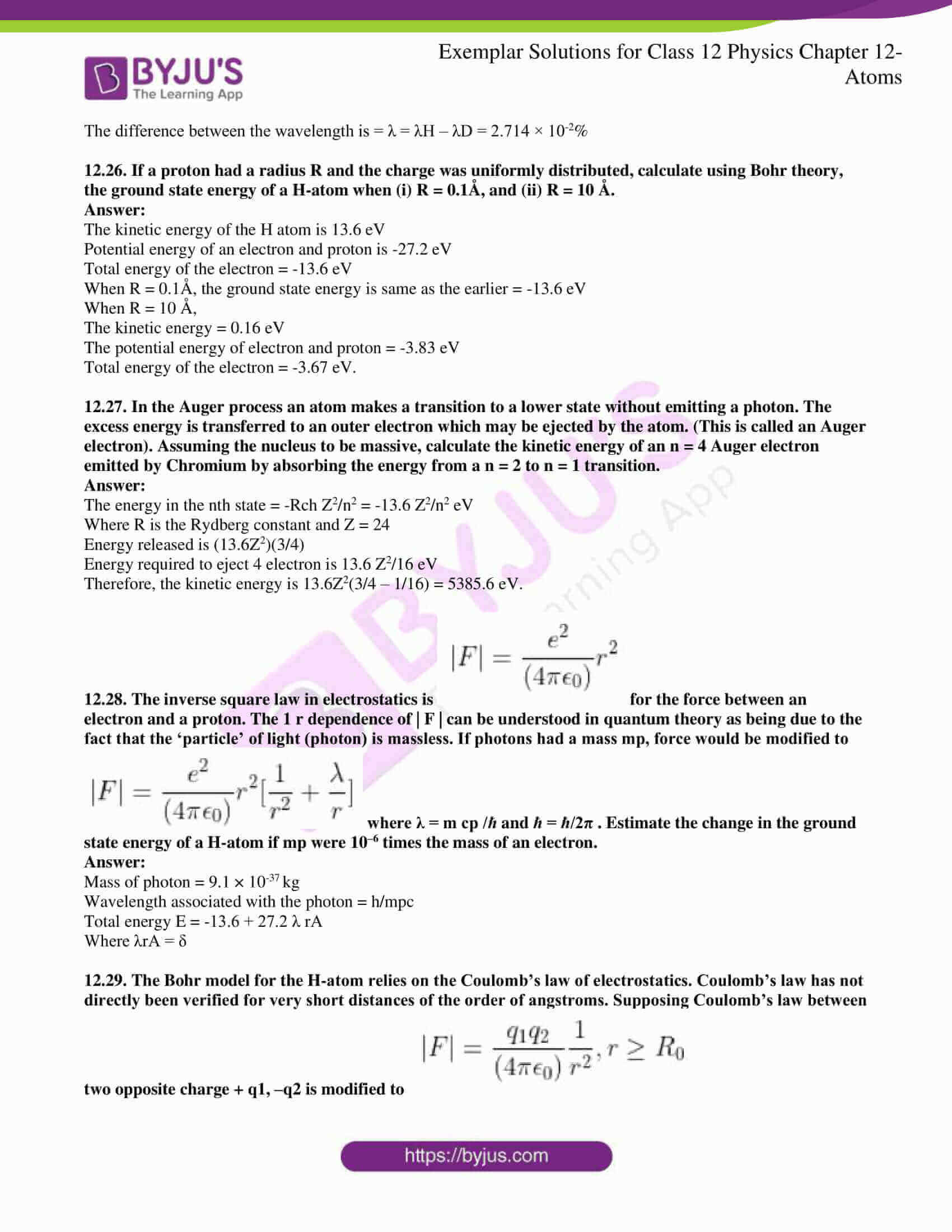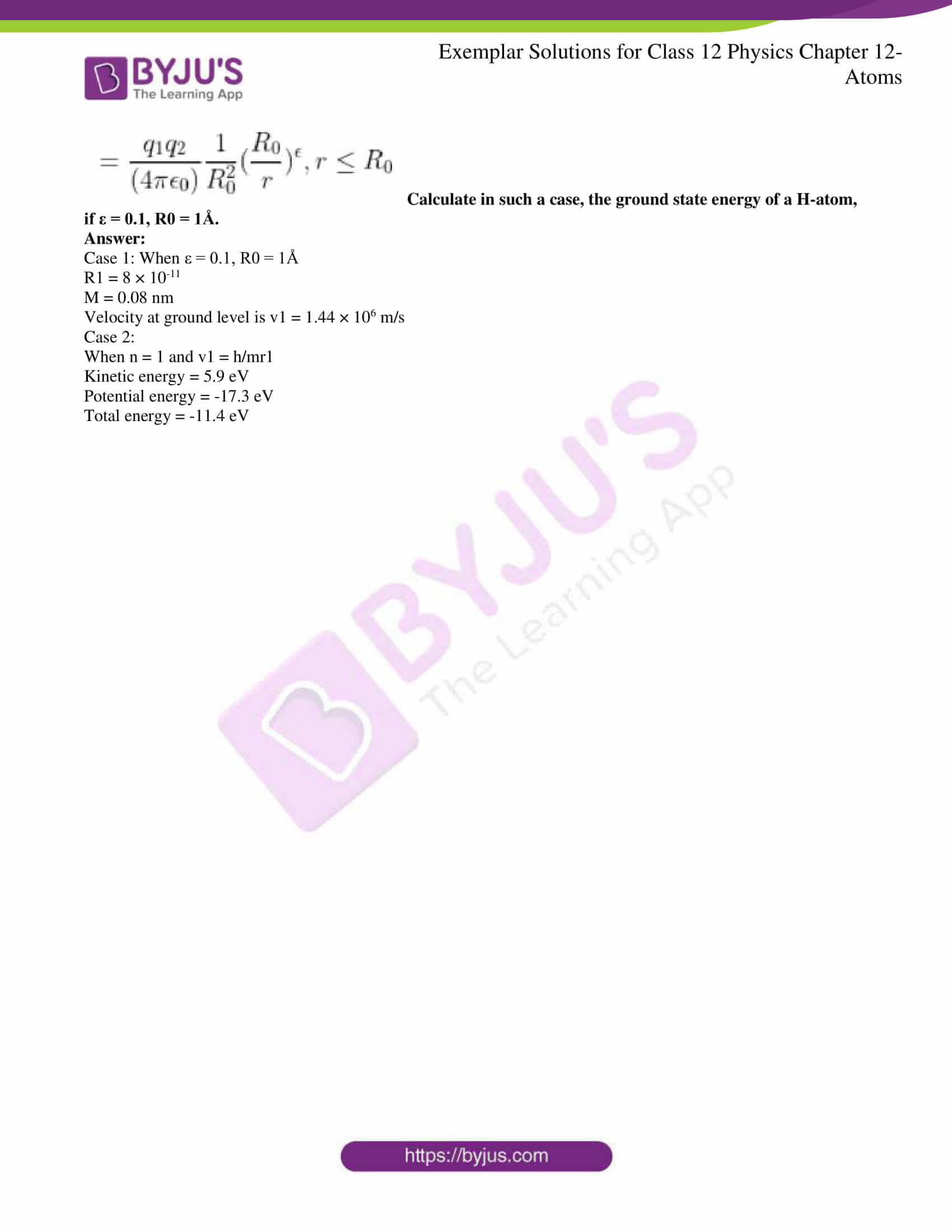### Multiple Choice Questions I

12.1. Taking the Bohr radius as a0 = 53pm, the radius of Li++ ion in its ground state, on the basis of Bohr’s model, will be about

(a) 53 pm

(b) 27 pm

(c) 18 pm

(d) 13 pm

(c) 18 pm

12.2. The binding energy of an H-atom, considering an electron moving around a fixed nucleus (proton), is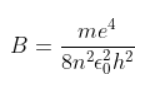(m = electron mass). If one decides to work in a frame of reference where the electron is at rest, the proton would be moving around it. By similar arguments, the binding energy would be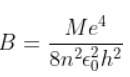(M = proton mass) This last expression is not correct because

(a) n would not be integral

(b) Bohr-quantisation applies only to electron

(c) the frame in which the electron is at rest is not inertial

(d) the motion of the proton would not be in circular orbits, even approximately

(c) the frame in which the electron is at rest is not inertial

12.3. The simple Bohr model cannot be directly applied to calculate the energy levels of an atom with many electrons. This is because

(a) of the electrons not being subject to a central force

(b) of the electrons colliding with each other

(c) of screening effects

(d) the force between the nucleus and an electron will no longer be given by Coulomb’s law

(a) of the electrons not being subject to a central force

12.4. For the ground state, the electron in the H-atom has an angular momentum = ℏ, according to the simple Bohr model. Angular momentum is a vector and hence there will be infinitely many orbits with the vector pointing in all possible directions. In actuality, this is not true,

(a) because Bohr model gives incorrect values of angular momentum

(b) because only one of these would have a minimum energy

(c) angular momentum must be in the direction of spin of electron

(d) because electrons go around only in horizontal orbits

(a) because Bohr model gives incorrect values of angular momentum

12.5. O2 molecule consists of two oxygen atoms. In the molecule, nuclear force between the nuclei of the two atoms

(a) is not important because nuclear forces are short-ranged

(b) is as important as an electrostatic force for binding the two atoms

(c) cancels the repulsive electrostatic force between the nuclei

(d) is not important because the oxygen nucleus have an equal number of neutrons and protons

(a) is not important because nuclear forces are short-ranged

12.6. Two H atoms in the ground state collide inelastically. The maximum amount by which their combined kinetic energy is reduced is

(a) 10.20 eV

(b) 20.40 eV

(c) 13.6 eV

(d) 27.2 eV

(a) 10.20 eV

12.7. A set of atoms in an excited state decays.

(a) in general to any of the states with lower energy

(b) into a lower state only when excited by an external electric field

(c) all together simultaneously into a lower state

(d) to emit photons only when they collide

(a) in general to any of the states with lower energy

### Multiple Choice Questions II

12.8. An ionised H-molecule consists of an electron and two protons. The protons are separated by a small distance of the order of angstrom. In the ground state,

(a) the electron would not move in circular orbits

(b) the energy would be (2)4 times that of an H-atom

(c) the electrons, the orbit would go around the protons

(d) the molecule will soon decay in a proton and an H-atom

(a) the electron would not move in circular orbits

(c) the electrons, the orbit would go around the protons

12.9. Consider aiming a beam of free electrons towards free protons. When they scatter, an electron and a proton cannot combine to produce an H-atom,

(a) because of energy conservation

(b) without simultaneously releasing energy in the form of radiation

(c) because of momentum conservation

(d) because of angular momentum conservation

(a) because of energy conservation

(b) without simultaneously releasing energy in the form of radiation

12.10. The Bohr model for the spectra of an H-atom

(a) will not be applicable to hydrogen in the molecular from

(b) will not be applicable as it is for a He-atom

(c) is valid only at room temperature

(d) predicts continuous as well as discrete spectral lines

(a) will not be applicable to hydrogen in the molecular from

(b) will not be applicable as it is for a He-atom

12.11 The Balmer series for the H-atom can be observed

(a) if we measure the frequencies of light emitted when an excited atom falls to the ground state

(b) if we measure the frequencies of light emitted due to transitions between excited states and the first excited state

(c) in any transition in a H-atom

(d) as a sequence of frequencies with the higher frequencies getting closely packed

(b) if we measure the frequencies of light emitted due to transitions between excited states and the first excited state

(d) as a sequence of frequencies with the higher frequencies getting closely packed

12.12. Let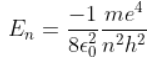be the energy of the nth level of H-atom. If all the H-atoms are in the ground state and radiation of frequency (E2-E1)/h falls on it

(a) it will not be absorbed at all

(b) some of the atoms will move to the first excited state

(c) all atoms will be excited to the n = 2 state

(d) no atoms will make a transition to the n = 3 state

(b) some of the atoms will move to the first excited state

(d) no atoms will make a transition to the n = 3 state

12.13. The simple Bohr model is not applicable to He4 atom because

(a) He4 is an inert gas

(b) He4 has neutrons in the nucleus

(c) He4 has one more electron

(d) electrons are not subject to central forces

(c) He4 has one more electron

(d) electrons are not subject to central forces

12.14. The mass of an H-atom is less than the sum of the masses of a proton and electron. Why is this?

We know that,

E = ∆mc2

The mass of the hydrogen atom is

mp + mp – BE/c2

Where BE = 13.6 eV.

12.15. Imagine removing one electron from He4 and He3. Their energy levels, as worked out on the basis of Bohr model will be very close. Explain why.

The energies of He4 and He3 will be very close according to the Bohr model because both have 1 electron as in case of the hydrogen atom and the nucleus is 4 times heavier than the H-atoms. Therefore, the stability of these atoms remains close to the H atom and so is their energy levels.

12.16. When an electron falls from a higher energy to a lower energy level, the difference in the energies appears in the form of electromagnetic radiation. Why cannot it be emitted as other forms of energy?

When an electron falls from a higher energy to a lower energy level, the difference in the energies appears in the form of electromagnetic radiation because there is an acceleration of the charged particle.

12.17. Would the Bohr formula for the H-atom remain unchanged if proton had a charge (+4/3)e and electron a charge (−3/4)e, where e = 1.6 × 10–19C? Give reasons for your answer.

As there is no change in the position of the proton and electron the product of both the charges will remain the same.

12.18. Consider two different hydrogen atoms. The electron in each atom is in an excited state. Is it possible for the electrons to have different energies but the same orbital angular momentum according to the Bohr model?

As the value of n in two different hydrogen atoms are different, their angular momentum will be different.

According to Bohr’s model, the angular momentum is given as

L = nh/2π

12.19. Positronium is just like an H-atom with the proton replaced by the positively charged anti-particle of the electron (called the positron which is as massive as the electron). What would be the ground state energy of positronium?

The lowest energy of the positronium is -6.8 electron volts

The highest energy level of the positronium which is the next to n = 1 that is n = 2 is -1.7 electron volt.

12.20. Assume that there is no repulsive force between the electrons in an atom but the force between positive and negative charges is given by Coulomb’s law as usual. Under such circumstances, calculate the ground state energy of a He-atom.

For He nucleus, Z = 2 and in-ground state n = 1

Therefore, En = -13.6 Z2/n2 eV = -54.4 eV

12.21. Using the Bohr model, calculate the electric current created by the electron when the H-atom is in the ground state.

Let v be the velocity of the electron

Number of revolutions per unit time = f = 2πa0/v

The electric current is given as I = Q

When change Q flows in time T

Such that Q = e

Therefore, the electric current is given as I = 2πa0/v e

12.22. Show that the first few frequencies of light that are emitted when electrons fall to the nth level from levels higher than n are approximate harmonics (i.e. in the ratio 1 : 2: 3…) when n >>1.

Frequency of any line in the series on the spectrum of hydrogen is expressed as the difference between the two atoms

fcm = cRZ2[1/(n+p)2 – 1/n2]

where m = n + p

Substituting for p we get,

fcm = (2cRZ2/n3)p

12.23. What is the minimum energy that must be given to an H atom in ground state so that it can emit an Hγ line in Balmer series? If the angular momentum of the system is conserved, what would be the angular momentum of such Hγ photon?

Energy required for the transition from n = 1 to n = 5 is given as:

E = E5 – E1 = (-13.6/52) – (-13.6/12) = 13.06 eV

Angular momentum is given as = change in angular momentum of electron = 5(h/2π) – 2(h/2 π) = 3h/2 π = 3.17 × 10-34 Js.

12.24. The first four spectral lines in the Lyman series of an H-atom are λ = 1218 Å, 1028Å, 974.3 Å and 951.4Å. If instead of Hydrogen, we consider Deuterium, calculate the shift in the wavelength of these lines.

Let μH and μD be the reduced masses of electrons of hydrogen and deuterium respectively.

ni and nf are the fixed mass series for hydrogen and deuterium

Rh/Rd = μH/ μD

Reduced mass of hydrogen = μH = me/1+me/M = me (1-me/M)

Reduced mass of deuterium = μD = 2M.me/2M(1+me/2M) = me(1- me/2M)

μH/ μD = 0.99973

λD/ λH = 0.99973

λD = 0.99973 λH

Therefore, change in wavelength = λH – λD = 0.3 Å

12.25. Deuterium was discovered in 1932 by Harold Urey by measuring the small change in wavelength for a particular transition in 1H and 2H. This is because the wavelength of transition depends to a certain extent on the nuclear mass. If nuclear motion is taken into account then the electrons and nucleus revolve around their common centre of mass. Such a system is equivalent to a single particle with a reduced mass µ, revolving around the nucleus at a distance equal to the electron-nucleus separation. Here µ = me M/(me +M) where M is the nuclear mass and me is the electronic mass. Estimate the percentage difference in wavelength for the 1st line of the Lyman series in 1H and 2H. (Mass of 1H nucleus is 1.6725 × 10–27 kg, Mass of 2H nucleus is 3.3374 × 10–27 kg, Mass of electron = 9.109 × 10–31 kg.)

The energy of an electron in the nth state is

En = -μZ2e4/8ε02h2(1/n2)

For hydrogen atom, μH = meMh/(me + Mh)

For deuterium atom, μD = meMd/(me + Md)

The wavelength of the transition for hydrogen is = λH = 3/4 μHe4/8ε02h2c

The wavelength of the transition for deuterium is = λD = 3/4 μDe4/8ε02h2c

The difference between the wavelength is = λ = λH – λD = 2.714 × 10-2%

12.26. If a proton had a radius R and the charge was uniformly distributed, calculate using Bohr theory, the ground state energy of a H-atom when (i) R = 0.1Å, and (ii) R = 10 Å.

The kinetic energy of the H atom is 13.6 eV

Potential energy of an electron and proton is -27.2 eV

Total energy of the electron = -13.6 eV

When R = 0.1Å, the ground state energy is same as the earlier = -13.6 eV

When R = 10 Å,

The kinetic energy = 0.16 eV

The potential energy of electron and proton = -3.83 eV

Total energy of the electron = -3.67 eV.

12.27. In the Auger process, an atom makes a transition to a lower state without emitting a photon. The excess energy is transferred to an outer electron which may be ejected by the atom. (This is called an Auger electron). Assuming the nucleus to be massive, calculate the kinetic energy of an n = 4 Auger electron emitted by Chromium by absorbing the energy from an = 2 to n = 1 transition.

The energy in the nth state = -Rch Z2/n2 = -13.6 Z2/n2 eV

Where R is the Rydberg constant and Z = 24

Energy released is (13.6Z2)(3/4)

Energy required to eject 4 electron is 13.6 Z2/16 eV

Therefore, the kinetic energy is 13.6Z2(3/4 – 1/16) = 5385.6 eV.

12.28. The inverse square law in electrostatics is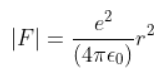for the force between an electron and a proton. The 1 r dependence of | F | can be understood in quantum theory as being due to the fact that the ‘particle’ of light (photon) is massless. If photons had a mass mp, force would be modified to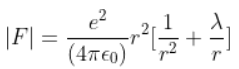where λ = m cp /ℏ and ℏ = ℏ/2π . Estimate the change in the ground state energy of an H-atom if mp were 10–6 times the mass of an electron.

Mass of photon = 9.1 × 10-37 kg

Wavelength associated with the photon = h/mpc

Total energy E = -13.6 + 27.2 λ rA

Where λrA = δ

12.29. The Bohr model for the H-atom relies on the Coulomb’s law of electrostatics. Coulomb’s law has not directly been verified for very short distances of the order of angstroms. Supposing Coulomb’s law between two opposite charge + q1, –q2 is modified to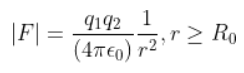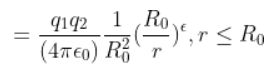Calculate in such a case, the ground state energy of an H-atom, if ε = 0.1, R0 = 1Å.

Case 1: When ε = 0.1, R0 = 1Å

R1 = 8 × 10-11

M = 0.08 nm

Velocity at ground level is v1 = 1.44 × 106 m/s

Case 2:

When n = 1 and v1 = h/mr1

Kinetic energy = 5.9 eV

Potential energy = -17.3 eV

Total energy = -11.4 eV

The basic unit of matter, which is the smallest thing in existence, is called an atom. The size of an atom is usually variable in nature, depending on the type of element. Protons, neutrons and electrons are the three different particles present inside the atom. Atoms, when grouped together, form molecules. For example, an oxygen molecule is made from smaller oxygen atoms which are grouped together.

The different parts of an atom are

1. Quarks
2. Proton
3. Neutron
4. Electron
5. Ion
6. Nucleus
7. Electrons

### Why Opt for BYJU’S

• The BYJU’S teaching methodology is unique that makes students fall in love with learning.
• The study materials we provide aid you in learning the concepts well and avoid the confusion you have in choosing the right study material.

Stay tuned to BYJU’S to get free notes, study materials, NCERT Solutions, previous years’ question papers, sample papers, worksheets and exemplar of all the subjects for all classes based on the CBSE syllabus of 2023-24.